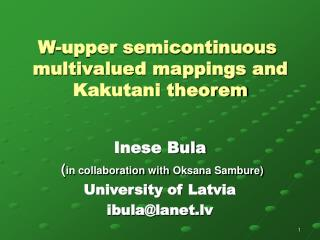DownloadDownload PresentationW-upper semicontinuous multivalued mappings and Kakutani theorem

# W-upper semicontinuous multivalued mappings and Kakutani theorem

Télécharger la présentation## W-upper semicontinuous multivalued mappings and Kakutani theorem

- - - - - - - - - - - - - - - - - - - - - - - - - - - E N D - - - - - - - - - - - - - - - - - - - - - - - - - - -
##### Presentation Transcript

1. W-upper semicontinuous multivalued mappings and Kakutani theorem Inese Bula (in collaboration withOksana Sambure) University of Latvia ibula@lanet.lv

2. Let X and Y be metric spaces. U(x,r) - open ball with center x and radius r. Let . Then is a neighbourhood of the set A. Definition 1.A multivalued mapping is called w-upper semicontinuous at a pointif If f is w-upper semicontinuous multivalued mapping for every point of space X, then such a mapping is called w-upper semicontinuous multivalued mapping in space X (or w-u.s.c.).

3. Every upper semicontinuous multivalued mapping is w-upper semicontinuous multivalued mapping (w>0) but not conversely. Example 1. and y 3 2 1 0 1 2 3 4 x This mapping is not upper semicontinuous multivalued mapping in point 2: But this mapping is 1-upper semicontinuous multivalued mapping in point 2. It is w-upper semicontinuous multivalued mapping in point 2 for every too.

4. We consider Definition 2.A multivalued mapping is called w-closed at a point x, if for all convergent sequences which satisfy it follows that If f is w-closed mapping for every point of space X, then such a mapping is called w-closed mapping in space X. In Example 1 considered function is 1-closed in point 2. It is w-closed mapping in point 2 for every too.

5. Let X, Y be normed spaces. We define a sum f + g of multivalued mappings as follows: We prove Theorem 1.If is w1-u.s.c. andis w2-u.s.c., then f + g is (w1+w2)-u.s.c. Corollary. If is w-u.s.c. andis u.s.c., then f + g is w-u.s.c.

6. Let X, Y be metric spaces. It is known for u.s.c.: • If K is compact subset of X and is compact-valued • u.s.c., then the set is compact. • If is compact-valued w-u.s.c., then it is possible that • is not compact even if K is compact subset of X. • Example 2. Suppose the mapping is y This mapping is compact-valued and 0.5-u.s.c., its domain is compact set [0,2], but this set is not compact, only bounded. 3 2.5 2.3 2 1 0 1 2 x

7. We prove Theorem 2.Let is compact-valued w-u.s.c. If is compact set, then is bounded set. In Example 1 considered mapping is 1-u.s.c., compact-valued and 1-closed. Is it regularity? We can observe: if mapping is w-closed, then it is possible that there is a point such that the image is not closed set. For example, Theorem 3. If multivalued mapping is w-u.s.c. and for every the image set f(x) is closed, then f is w-closed.

8. Analog of Kakutani theorem Theorem 4.Let K be a compact convex subset of normed space X. Let be a w-u.s.c. multivalued mapping. Assume that for every , the image f(x) is a convex closed subset of K. Then there exists such that , that is B(x,r) - closed ball with center x and radius r.

9. Idea of PROOF. We define mapping This mapping satisfies the assumptions of the Kakutani theorem: If C be a compact convex subset of normed space X and if be a closed and convex-valued multivalued mapping, then there exists at least one fixed point of mapping f. Then It follows (f is w-u.s.c. multivalued mapping!) Therefore

10. In one-valued mapping case we have: Definition 1.A mapping is called w-continuous at a point if If f is w-continuous mapping for every point of space X, then such a mapping is called w-continuous mapping in space X . Corollary. Let K be a compact convex subset of normed space X. Let is w-continuous mapping. Then

11. References • I.Bula, Stability of the Bohl-Brouwer-Schauder theorem, Nonlinear Analysis, Theory, Methods & Applications, V.26, P.1859-1868, 1996. • M.Burgin, A. Šostak, Towards the theory of continuity defect and continuity measure for mappings of metric spaces, Latvijas Universitātes Zinātniskie Raksti, V.576, P.45-62, 1992. • M.Burgin, A. Šostak, Fuzzyfication of the Theory of Continuous Functions, Fuzzy Sets and Systems, V.62, P.71-81, 1994. • O.Zaytsev, On discontinuous mappings in metric spaces, Proc. of the Latvian Academy of Sciences, Section B, v.52, 259-262, 1998.

12. Thank You!